# rolerman-AnimatedLoop

My final design is an RGB deconstruction/reconstruction GIF: a square travels in a path around the screen, falling apart into its separate RGB channels along the way.

My GIF: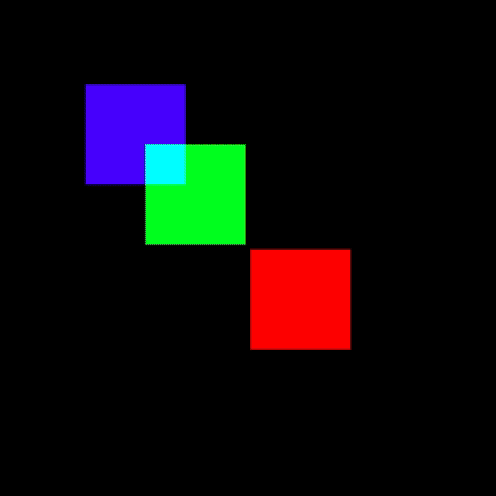Graphical Concept

I struggled to come up with a concept for this assignment, and found myself drawing a blank when it came to ideas. I looked at a bunch of Saskia Freeke and Bees & Bombs to help get my brain started, but it took a while for me to begin to have ideas of my own. My favorite moment was when I began to think of digital shapes as being composed of other shapes: particularly, my primary concept became showing that the color white is composed out of every other color. The easing function I chose was a quadratic ease-in-out, because I liked the mild snap to it.

My main personal critique is that I’m not entirely happy with the “punch” of it. I wish it had wound up looking more interesting, and I’m frustrated that the visual intrigue isn’t there as much as I wanted it to be. However, I do think it is satisfying to watch, and I enjoy the fact that it illustrates physical properties of light.

Inspiration

Thank you to Bees & Bombs for the inspiration. Some of my favorite examples on his blog involved deconstructing shapes into their separate RGB channels, and dealing with overlapping spaces, and this was my main inspiration.

By Bees & Bombs: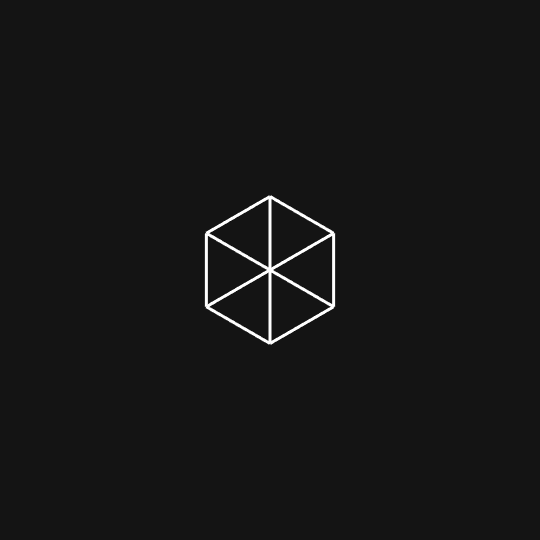By Bees & Bombs: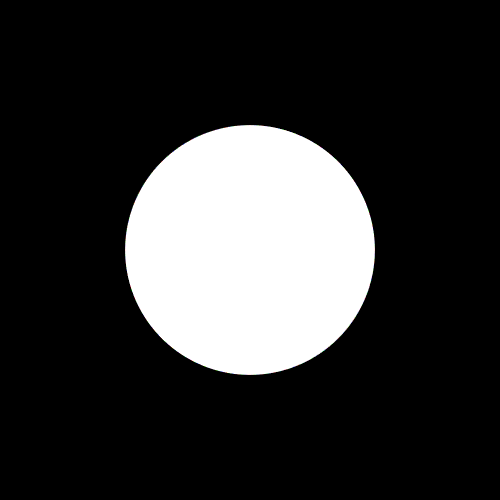Technical Implementation

My biggest challenges in creating my GIF were:

1. Getting the squares to travel in a path
2. Having each square run at a delay
3. Calculating the intersections

Getting the squares to travel in a path

I took the “percent” parameter in the template, and converted that to a “phase” between 0 and 3. The squares travel in a path around the page. When they are in phase 0, they are moving towards point 1. When they are in phase 1, they are moving toward point 2. Depending on how far along we are in the current phase, that is how far along its path the square travels.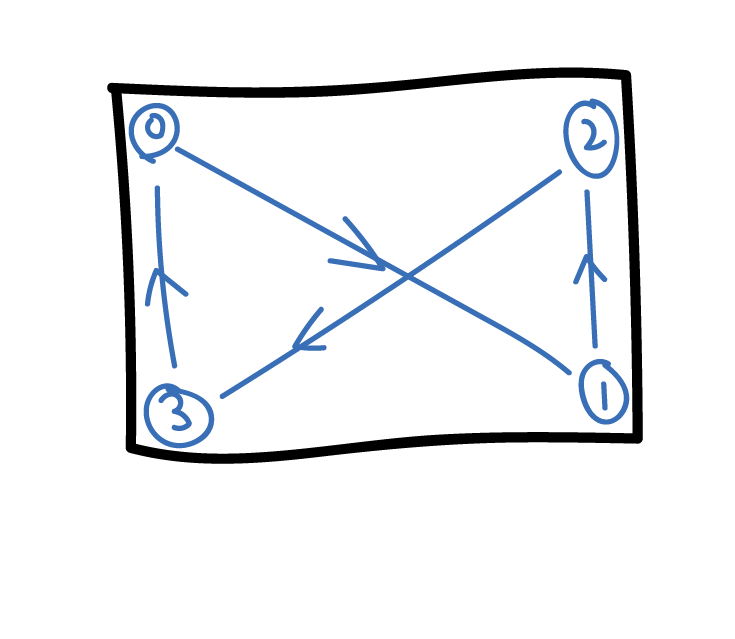Having each square run at a delay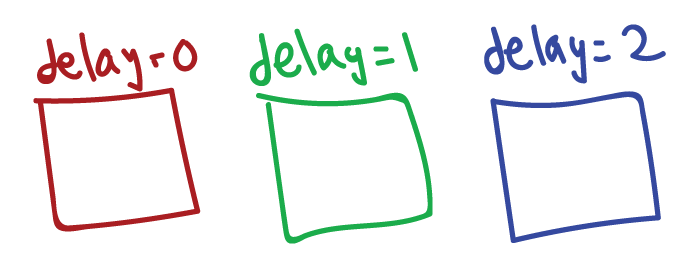Each square has a “delay” property, and this is factored in into whether it moves or not. This actually wound up giving me a ton of crazy bugs, where it would jump a bunch after waiting the expected delay time. I wound up multiplying the percentage of phase completion by a certain value to combat this.

Calculating the intersections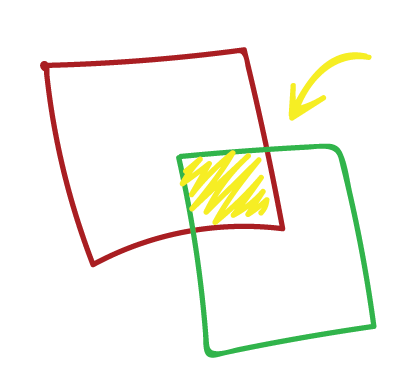While this should have been simple math, it wound up breaking my brain a bit, since it’s different depending on which direction the squares are coming from.

First prototype

This is a GIF from when I first figured out how to get the intersections to work, without any of the path following:Full brainstorm

For more scatterbrained documentation, please refer to the whiteboard wall I covered in pursuit of this project: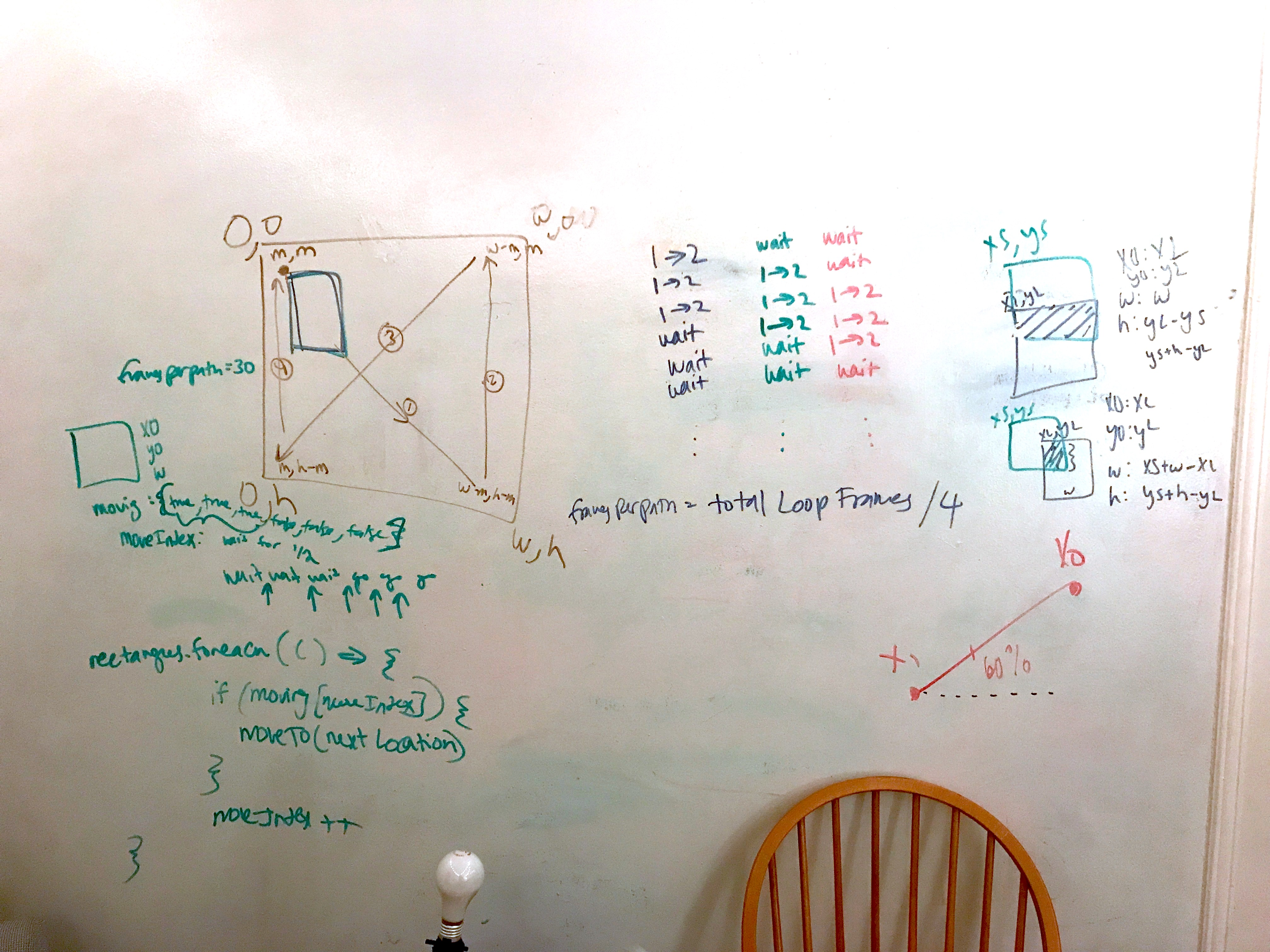Code

```// All of the code is an implementation of Golan Levin's // Loop templates: https://github.com/golanlevin/LoopTemplates     // ========= Golan's template functions ============   // User-modifiable global variables. var myNickname = "rolerman"; var nFramesInLoop = 240; var bEnableExport = true;   // Other global variables you don't need to touch. var nElapsedFrames; var bRecording; var theCanvas; const canvasW = 500;   function setup() { theCanvas = createCanvas(canvasW, canvasW); bRecording = false; nElapsedFrames = 0; }   function keyTyped() { if (bEnableExport) { if ((key === 'f') || (key === 'F')) { bRecording = true; nElapsedFrames = 0; } } }   function draw() {   // Compute a percentage (0...1) representing where we are in the loop. var percentCompleteFraction = 0; if (bRecording) { percentCompleteFraction = float(nElapsedFrames) / float(nFramesInLoop); } else { percentCompleteFraction = float(frameCount % nFramesInLoop) / float(nFramesInLoop); }   // Render the design, based on that percentage. // This function renderMyDesign() is the one for you to change. renderMyDesign (percentCompleteFraction);   // If we're recording the output, save the frame to a file. // Note that the output images may be 2x large if you have a Retina mac. // You can compile these frames into an animated GIF using a tool like: if (bRecording && bEnableExport) { var frameOutputFilename = myNickname + "_frame_" + nf(nElapsedFrames, 4) + ".png"; print("Saving output image: " + frameOutputFilename); saveCanvas(theCanvas, frameOutputFilename, 'png'); nElapsedFrames++;   if (nElapsedFrames >= nFramesInLoop) { bRecording = false; } } }     // from R. Luke Dubois' p5-func: // https://github.com/IDMNYU/p5.js-func const quadraticInOut = function(_x) { if(_x < 0.5) { return(2 * _x * _x); } else { return((-2 * _x * _x) + (4 * _x) - 1); } }     // ========= My code starts here ============   // Rectangle class stores all necessary rectangle data   class Rectangle { constructor(x0, y0, w, h, moves, clr, delay) { this.x0 = x0; this.y0 = y0; this.w = w; this.h = h; this.moves = moves; this.moveIndex = 0; this.color = clr; this.percentThroughPath = 0; this.delay = delay; }   moveTo(x0, y0, x1, y1) { let percentOff = percentThroughPhase - this.delay / 2.0 if (percentOff < 0) { return; } if (percentOff > 1) { return; } const easedPercentOff = quadraticInOut(percentOff) this.x0 = (x1 - x0) * (easedPercentOff) + x0 this.y0 = (y1 - y0) * (easedPercentOff) + y0 }   drawSelf() { fill(this.color); rect(this.x0, this.y0, this.w, this.w); } }     // given two rectangles, calculate the color of their intersection // (naive, this implementation only works for pure R, G, B) function getIntersectingColor(rect1, rect2) { let newColor = "#" for (let i = 1; i < rect1.color.length; i++) { if (rect1.color[i] === "0") newColor += rect2.color[i]; else newColor += rect1.color[i]; } return newColor; }   // global structures for the intersection squares yellow = { x0:0, y0:0, w: 0, h: 0, color: "#ffff00" } cyan = { x0:0, y0:0, w: 0, h: 0, color: "#00ffff" } magenta = { x0:0, y0:0, w: 0, h: 0, color: "#ff00ff" }   // calculate the rectangle intersection of two rectangles function rectIntersect(rect1, rect2) { const clr = getIntersectingColor(rect1, rect2); let xs, xl; let ys, yl; const w = Math.min(rect2.w, rect1.w); const h = Math.min(rect2.h, rect1.h); if (rect1.x0 < rect2.x0) { xs = rect1.x0; xl = rect2.x0; } else { xs = rect2.x0; xl = rect1.x0; }   if (rect1.y0 < rect2.y0) { ys = rect1.y0; yl = rect2.y0; } else { ys = rect2.y0; yl = rect1.y0; }   if (((xs + w) < xl )|| ((ys + h) < yl)) { return; }   const x0 = xl; const y0 = yl; let wid; let hei; wid = (xs + w) - xl; hei = ys + h - yl;   // update global intersect objects with newly calculated coordinates if (clr === "#ffff00") { yellow = { x0, y0, w: wid, h: hei, color: "#ffff00" } } else if (clr === "#00ffff") { cyan = { x0, y0, w: wid, h: hei, color: "#00ffff" } } else if (clr === "#ff00ff") { magenta = { x0, y0, w: wid, h: hei, color: "#ff00ff" } } fill(clr) if (clr === "#ffffff") { rect(x0, y0, wid , hei); } }   let currentPhase = 0; const margin = 80; const squareW = 100;   // the four points that the squares chase around the screen const phaseCoords = [ { x: canvasW - margin - squareW, y: margin }, { x: margin, y: canvasW - margin - squareW }, { x: margin, y: margin }, { x: canvasW - margin - squareW, y: canvasW - margin - squareW } ]     // initial R, G, and B rectangles   let rectangles = [] rectangles.push(new Rectangle( phaseCoords.x, phaseCoords.y, squareW, squareW, [true, true, true, false, false], "#ff0000", 0) ) rectangles.push(new Rectangle( phaseCoords.x, phaseCoords.y, squareW, squareW, [false, true, true, true, false], "#00ff00", 0.5) ) rectangles.push(new Rectangle( phaseCoords.x, phaseCoords.y, squareW, squareW, [false, false, true, true, true], "#0000ff", 1) )   let percentThroughPhase; let drawWhite = true;   function renderMyDesign (percent) { background(0); strokeWeight(0);   // are we in phase 0, 1, 2, or 3? // how far are we through that phase?   if (percent <= 0.25) { currentPhase = 0; percentThroughPhase = percent * 4; } else if (percent <= 0.5) { currentPhase = 1; percentThroughPhase = (percent - 0.25) * 4; } else if (percent <= 0.75) { currentPhase = 2; percentThroughPhase = (percent - 0.5) * 4; } else { currentPhase = 3; percentThroughPhase = (percent - 0.75) * 4; }   // multiply by this offset due to the delays of the squares percentThroughPhase *= 3.0/2.0;     // move every rectangle towards its next destination rectangles.forEach((R) => { R.moveTo( phaseCoords[currentPhase].x, phaseCoords[currentPhase].y, phaseCoords[(currentPhase + 1) % 4].x, phaseCoords[(currentPhase + 1) % 4].y ) })   // draw every rectangle rectangles.drawSelf(); rectangles.drawSelf(); rectangles.drawSelf();   // calculate every intersection for (let i = 0; i < rectangles.length - 1; i++) { rectIntersect(rectangles[i], rectangles[i+1]); }   // draw every intersection fill(yellow.color); rect(yellow.x0, yellow.y0, yellow.w, yellow.h);   fill(cyan.color); rect(cyan.x0, cyan.y0, cyan.w, cyan.h);   fill(magenta.color); rect(magenta.x0, magenta.y0, magenta.w, magenta.h);     // draw the final layer of intersection (white) rectIntersect(yellow, cyan); rectIntersect(cyan, magenta); rectIntersect(yellow, magenta); }```